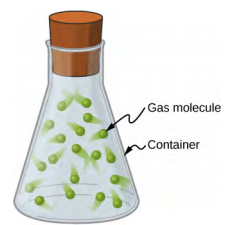# 9.2: Linear Momentum

•• OpenStax
• OpenStax
$$\newcommand{\vecs}{\overset { \rightharpoonup} {\mathbf{#1}} }$$ $$\newcommand{\vecd}{\overset{-\!-\!\rightharpoonup}{\vphantom{a}\smash {#1}}}$$$$\newcommand{\id}{\mathrm{id}}$$ $$\newcommand{\Span}{\mathrm{span}}$$ $$\newcommand{\kernel}{\mathrm{null}\,}$$ $$\newcommand{\range}{\mathrm{range}\,}$$ $$\newcommand{\RealPart}{\mathrm{Re}}$$ $$\newcommand{\ImaginaryPart}{\mathrm{Im}}$$ $$\newcommand{\Argument}{\mathrm{Arg}}$$ $$\newcommand{\norm}{\| #1 \|}$$ $$\newcommand{\inner}{\langle #1, #2 \rangle}$$ $$\newcommand{\Span}{\mathrm{span}}$$ $$\newcommand{\id}{\mathrm{id}}$$ $$\newcommand{\Span}{\mathrm{span}}$$ $$\newcommand{\kernel}{\mathrm{null}\,}$$ $$\newcommand{\range}{\mathrm{range}\,}$$ $$\newcommand{\RealPart}{\mathrm{Re}}$$ $$\newcommand{\ImaginaryPart}{\mathrm{Im}}$$ $$\newcommand{\Argument}{\mathrm{Arg}}$$ $$\newcommand{\norm}{\| #1 \|}$$ $$\newcommand{\inner}{\langle #1, #2 \rangle}$$ $$\newcommand{\Span}{\mathrm{span}}$$$$\newcommand{\AA}{\unicode[.8,0]{x212B}}$$

## Learning Objectives

• Explain what momentum is, physically
• Calculate the momentum of a moving object

Our study of kinetic energy showed that a complete understanding of an object’s motion must include both its mass and its velocity

$K = \left(\dfrac{1}{2}\right)mv^2.$

However, as powerful as this concept is, it does not include any information about the direction of the moving object’s velocity vector (e.g. the ball in Figure $$\PageIndex{1}$$). We’ll now define a physical quantity that includes direction.Figure $$\PageIndex{1}$$: The velocity and momentum vectors for the ball are in the same direction. The mass of the ball is about 0.5 kg, so the momentum vector is about half the length of the velocity vector because momentum is velocity time mass. (credit: modification of work by Ben Sutherland)

Like kinetic energy, this quantity includes both mass and velocity; like kinetic energy, it is a way of characterizing the “quantity of motion” of an object. It is given the name momentum (from the Latin word movimentum, meaning “movement”), and it is represented by the symbol $$p$$.

## Definition: Momentum

The linear momentum $$p$$ of an object is the product of its mass and its velocity:

$\vec{p} = m \vec{v} \ldotp \label{9.1}$

As shown in Figure $$\PageIndex{1}$$, momentum is a vector quantity (since velocity is). This is one of the things that makes momentum useful and not a duplication of kinetic energy. It is perhaps most useful when determining whether an object’s motion is difficult to change (Figure $$\PageIndex{1}$$) or easy to change (Figure $$\PageIndex{2}$$).Figure $$\PageIndex{2}$$: This supertanker transports a huge mass of oil; as a consequence, it takes a long time for a force to change its (comparatively small) velocity. (credit: modification of work by “the_tahoe_guy”/Flickr)

Unlike kinetic energy, momentum depends equally on an object’s mass and velocity. For example, as you will learn when you study thermodynamics, the average speed of an air molecule at room temperature (Figure $$\PageIndex{3}$$) is approximately 500 m/s, with an average molecular mass of $$6 \times 10^{−25}\, kg$$; its momentum is thus

\begin{align*} p_{molecule} &= (6 \times 10^{-25}\; kg)(500\; m/s) \\[4pt] &= 3 \times 10^{-22}\; kg\; \cdotp m/s \ldotp \end{align*}

For comparison, a typical automobile might have a speed of only 15 m/s, but a mass of 1400 kg, giving it a momentum of

\begin{align*} p_{car} &= (1400\; kg)(15\; m/s) \\[4pt] &= 21,000\; kg\; \cdotp m/s \ldotp \end{align*}

These momenta are different by 27 orders of magnitude, or a factor of a billion billion billion!Figure $$\PageIndex{3}$$: Gas molecules can have very large velocities, but these velocities change nearly instantaneously when they collide with the container walls or with each other. This is primarily because their masses are so tiny.

This page titled 9.2: Linear Momentum is shared under a CC BY license and was authored, remixed, and/or curated by OpenStax.